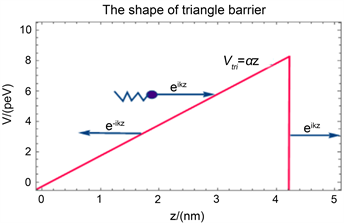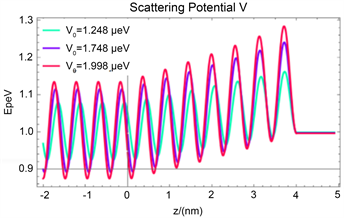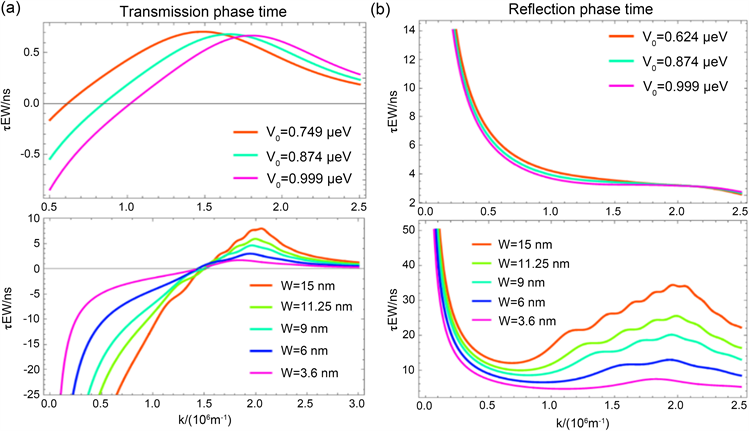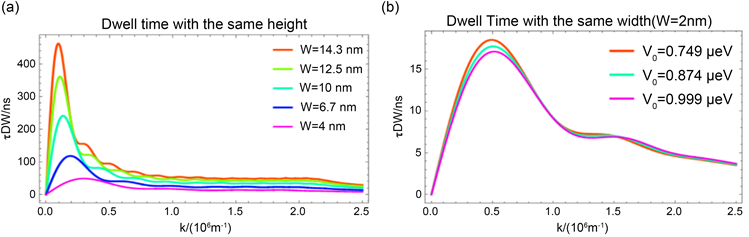# 三角势垒下的隧穿时间The Tunnel Time in a Triangular Potential

DOI: 10.12677/AAM.2021.104130, PDF, HTML, XML, 下载: 13  浏览: 39

Abstract: Quantum tunneling is a pure quantum phenomenon without any classical analogy. Whether it is instantaneous process or a process with finite time is an intensively debated issue since the early days of quantum mechanics. In this paper, we calculate the Wigner phase time and dwell time for a particle tunneling through a triangle barrier. We find that with the change of wave number k, the transmission phase time and the reflection phase show two opposite trends, while the dwell time changes the same as the transmission phase time. However, the phase time and dwell time both appear peak and oscillate.

1. 引言

2. 三角势垒下薛定谔方程的解

${V}_{tri}=\left\{\begin{array}{l}0\text{\hspace{0.17em}}\text{\hspace{0.17em}}\text{\hspace{0.17em}}\text{\hspace{0.17em}}\text{\hspace{0.17em}}z<0或z\ge l\\ \alpha z\text{\hspace{0.17em}}\text{\hspace{0.17em}}\text{\hspace{0.17em}}\text{\hspace{0.17em}}\text{\hspace{0.17em}}0\le z (1)Figure 1. Particles pass through a triangular barrier

$i\hslash \frac{\partial }{\partial t}\Psi \left(z,t\right)=\left[-\frac{{\hslash }^{2}}{2m}+V\left(z\right)\right]\Psi \left(z,t\right)$ (2)

${{\psi }^{″}}_{E}\left(z\right)+\frac{2mE}{{\hslash }^{2}}\left(1-\frac{V\left(z\right)}{E}\right){\psi }_{E}\left(z\right)=0$ (3)

${\psi }_{I}={\text{e}}^{ikz}+R{\text{e}}^{-ikz},\text{\hspace{0.17em}}\text{\hspace{0.17em}}\text{\hspace{0.17em}}z<0$ (4)

${\psi }_{II}={c}_{1}\text{Ai}\left[-{E}_{D}+{z}_{D}\right]+{c}_{2}\text{Bi}\left[-{E}_{D}+{z}_{D}\right],\text{\hspace{0.17em}}\text{\hspace{0.17em}}\text{\hspace{0.17em}}0\le z (5)

${\psi }_{III}=T{\text{e}}^{-ikz},\text{\hspace{0.17em}}\text{\hspace{0.17em}}\text{\hspace{0.17em}}l\le z$ (6)

$1+R={c}_{1}\text{Ai}\left[-{E}_{D}\right]+{c}_{2}\text{Bi}\left[-{E}_{D}\right]$ (7)

$ik\left(1-R\right)=\frac{1}{{L}_{C}}\left({c}_{1}Ai\prime \left[-{E}_{D}\right]+{c}_{2}B{i}^{\prime }\left[-{E}_{D}\right]\right)$ (8)

$T{\text{e}}^{ikl}={c}_{1}\text{Ai}\left[{l}_{d}-{E}_{D}\right]+{c}_{2}\text{Bi}\left[{l}_{d}-{E}_{D}\right]$ (9)

$ikT{\text{e}}^{ikl}=\frac{1}{{L}_{C}}\left({c}_{1}Ai\prime \left[{l}_{d}-{E}_{D}\right]+{c}_{2}B{i}^{\prime }\left[{l}_{d}-{E}_{D}\right]\right)$ (10)

$R=1+\frac{-2+2ik{L}_{C}P}{M+k{L}_{C}\left(iF+k{L}_{C}Q\right)};T=\frac{2i{\text{e}}^{-ikl}Jk{L}_{C}}{M+k{L}_{C}\left(iF+k{L}_{C}Q\right)}$

${c}_{1}=-\frac{2k{L}_{C}\left(iB{i}^{\prime }\left[{l}_{d}-{E}_{D}\right]+\text{Bi}\left[{l}_{d}-{E}_{D}\right]k{L}_{C}\right)}{M+k{L}_{C}\left(iF+k{L}_{C}Q\right)}$ (11)

${c}_{2}=\frac{2k{L}_{C}\left(iAi\prime \left[{l}_{d}-{E}_{D}\right]+\text{Ai}\left[{l}_{d}-{E}_{D}\right]k{L}_{C}\right)}{M+k{L}_{C}\left(iF+k{L}_{C}Q\right)}$

(7)~(10)式中的M、P、Q、J、F分别为：

$M=Ai\prime \left[-{E}_{D}+{l}_{d}\right]B{i}^{\prime }\left[-{E}_{D}\right]-Ai\prime \left[-{E}_{D}\right]B{i}^{\prime }\left[-{E}_{D}+{l}_{d}\right]$

$P=-\text{Bi}\left[-{E}_{D}+{l}_{d}\right]A{i}^{\prime }\left[-{E}_{D}\right]+\text{Ai}\left[-{E}_{D}+{l}_{d}\right]B{i}^{\prime }\left[-{E}_{D}\right]$

$Q=\text{Ai}\left[-{E}_{D}+{l}_{d}\right]\text{Bi}\left[-{E}_{D}\right]-\text{Ai}\left[-{E}_{D}\right]\text{Bi}\left[-{E}_{D}+{l}_{d}\right]$ (12)

$J=\text{Bi}\left[-{E}_{D}+{l}_{d}\right]A{i}^{\prime }\left[-{E}_{D}+{l}_{d}\right]-\text{Ai}\left[-{E}_{D}+{l}_{d}\right]B{i}^{\prime }\left[-{E}_{D}+{l}_{d}\right]$

$\begin{array}{c}F=\text{Bi}\left[-{E}_{D}+{l}_{d}\right]A{i}^{\prime }\left[-{E}_{D}\right]+\text{Bi}\left[-{E}_{D}\right]A{i}^{\prime }\left[-{E}_{D}+{l}_{d}\right]\\ \text{\hspace{0.17em}}-\text{Ai}\left[-{E}_{D}+{l}_{d}\right]B{i}^{\prime }\left[-{E}_{D}\right]-\text{Ai}\left[-{E}_{D}\right]B{i}^{\prime }\left[-{E}_{D}+{l}_{d}\right]\end{array}$Figure 2. Wave function modulo as a function of z

3. 三角势垒的相位时间

$T\left(k\right){\text{e}}^{i\left[\alpha \left(k\right)+kx-E\left(k\right)t/\hslash \right]}$ (13)

$R\left(k\right){\text{e}}^{i\left[\beta \left(k\right)+kx-E\left(k\right)t/\hslash \right]}$ (14)

$\frac{\text{d}\alpha }{\text{d}k}+{x}_{p}\left(t\right)-\frac{1}{\hslash }\frac{\text{d}E}{\text{d}k}t=0$ (15)

$\delta {\tau }_{T}^{P}=\hslash \frac{\text{d}\alpha }{\text{d}E}=\frac{1}{v\left(k\right)}\frac{\text{d}\alpha }{\text{d}k}$ (16)

$\delta {\tau }_{R}^{P}=\hslash \frac{\text{d}\beta }{\text{d}E}=\frac{1}{v\left(k\right)}\frac{\text{d}\beta }{\text{d}k}$ (17)

${\tau }_{T}^{P}=\frac{-{g}^{2}\left({F}^{2}{k}^{2}l+FgM+{g}^{2}l{M}^{2}+2F{k}^{2}lP\right)+Fg{k}^{2}Q+{k}^{4}l{Q}^{2}}{{F}^{2}{g}^{2}{k}^{3}+k{\left({g}^{2}M+{k}^{2}Q\right)}^{2}}$ (18)

$\begin{array}{c}H=\text{Bi}\left[-{E}_{D}+{l}_{d}\right]A{i}^{\prime }\left[-{E}_{D}\right]-\text{Bi}\left[-{E}_{D}\right]A{i}^{\prime }\left[-{E}_{D}+{l}_{d}\right]\\ \text{\hspace{0.17em}}-\text{Ai}\left[-{E}_{D}+{l}_{d}\right]B{i}^{\prime }\left[-{E}_{D}\right]+\text{Ai}\left[-{E}_{D}\right]B{i}^{\prime }\left[-{E}_{D}+{l}_{d}\right]\end{array}$

$G=\text{Bi}\left[-{E}_{D}\right]A{i}^{\prime }\left[-{E}_{D}+{l}_{d}\right]-\text{Ai}\left[-{E}_{D}\right]B{i}^{\prime }\left[-{E}_{D}+{l}_{d}\right]$

${q}_{1}=iFgk-{g}^{2}M-{k}^{2}Q$ , ${q}_{2}=-iFgk-{g}^{2}M-{k}^{2}Q$ (19)

${q}_{3}=-gM+ikP$ , ${q}_{4}=-gM-ikP$

${q}_{5}=-\frac{{k}^{2}}{{g}^{3}}+l$ , ${q}_{6}=-\frac{2{k}^{2}}{{g}^{4}}+\frac{2l}{g}$

$\begin{array}{c}{\tau }_{R}^{p}=\left(i\left(1-\frac{2g{q}_{4}}{{q}_{2}}\right)\left(-{q}_{2}\left({q}_{2}-2g{q}_{4}\right)\left(-2i{k}^{4}Q{q}_{1}-2{g}^{2}{k}^{2}\left(-iM{q}_{1}+\left(G+H\right)k{q}_{3}\right)\\ \text{\hspace{0.17em}}\text{ }\text{ }+2g{k}^{3}\left(G{q}_{1}+ikQ{q}_{3}\right)-{g}^{4}\left(2kQ{q}_{3}+P{q}_{1}\left(i+2k{q}_{5}\right)\right)+{g}^{6}kP{q}_{3}{q}_{6}\\ \text{ }\text{ }\text{ }\text{\hspace{0.17em}}-i{g}^{5}{q}_{3}\left(F-{k}^{2}Q{q}_{6}\right)\right)+{q}_{1}\left({q}_{1}-2g{q}_{3}\right)\left(2i{k}^{4}Q{q}_{2}-2{g}^{2}{k}^{2}\left(iM{q}_{2}+\left(G+H\right)k{q}_{4}\right)\\ \text{\hspace{0.17em}}\text{ }\text{ }\text{ }+2g{k}^{3}\left(G{q}_{2}-ikQ{q}_{4}\right)+{g}^{4}\left(-2kQ{q}_{4}+P{q}_{2}\left(i-2k{q}_{5}\right)\right)\\ \begin{array}{c}{}_{}{}^{}\\ \end{array}+{g}^{6}kP{q}_{4}{q}_{7}+i{g}^{5}{q}_{4}\left(F-{k}^{2}Q{q}_{6}\right)\right)\right)\right)/\left({g}^{3}{q}_{1}\left(k{q}_{1}-2gk{q}_{3}\right){\left({q}_{2}-2g{q}_{4}\right)}^{2}\right)\end{array}$ (20)注：(a)图中的顶部是粒子在势垒宽度均为2 nm相同时，不同势垒高度下的透射相位时间；(a)图底部为势垒高度(1.13 μev)一致时，不同势垒宽度下的透射相位时间。(b)图中顶部是粒子在势垒宽度相同均为2 nm时，不同势垒高度下的反射相位时间；(b)图底部为势垒高度(1.13 μev)一致时，不同势垒宽度下的反射相位时间。

Figure 3. Phase time as a function of wave number kTable 1. Transmission time peaks and corresponding k values at different heights with the same barrier widthsTable 2. Transmission time peaks and corresponding k values at different width with the same barrier height

4. 三角势垒的驻留时间

${\tau }_{D}\left({x}_{1},{x}_{2},k\right)=\frac{1}{j\left(k\right)}{\int }_{{x}_{1}}^{{x}_{2}}\text{d}x{|\Psi \left(x,k\right)|}^{2}$ (21)

$\begin{array}{c}{\tau }_{D}=\frac{1}{k}\left({l}_{1}+{l}_{1}\left(1-\frac{2g{q}_{3}}{{q}_{1}}\right)\left(1-\frac{2g{q}_{4}}{{q}_{2}}\right)+\frac{{\text{e}}^{-ik{l}_{1}}\left(1+\frac{{\text{e}}^{2ik{l}_{1}}\left({q}_{1}-2g{q}_{3}\right)}{{q}_{1}}-\frac{2g{q}_{4}}{{q}_{2}}\right)\mathrm{sin}\left[k{l}_{1}\right]}{k}\\ \text{\hspace{0.17em}}-\frac{4{g}^{2}{k}^{2}\left(l-{l}_{2}\right){\left(\text{Bi}\left[{l}_{d}-{E}_{D}\right]A{i}^{\prime }\left[{l}_{d}-{E}_{D}\right]-\text{Ai}\left[{l}_{d}-{E}_{D}\right]B{i}^{\prime }\left[{l}_{d}-{E}_{D}\right]\right)2}^{}}{{F}^{2}{g}^{2}{k}^{2}+{\left({g}^{2}M+{k}^{2}Q\right)}^{2}}\\ \text{\hspace{0.17em}}-\frac{8}{Ecg{q}_{1}{q}_{2}}\left(E\text{Ai}\left[-{E}_{D}\right]\text{Bi}\left[-{E}_{D}\right]-E\text{Ai}\left[{l}_{d}-{E}_{D}\right]\text{Bi}\left[{l}_{d}-{E}_{D}\right]+Ecgl\text{Ai}\left[{l}_{d}-{E}_{D}\right]\text{Bi}\left[{l}_{d}-{E}_{D}\right]\\ \text{\hspace{0.17em}}+EcAi\prime \left[-{E}_{D}\right]B{i}^{\prime }\left[-{E}_{D}\right]-EcAi\prime \left[{l}_{d}-{E}_{D}\right]B{i}^{\prime }\left[{l}_{d}-{E}_{D}\right]\right)\left({k}^{4}\text{Ai}\left[{l}_{d}-{E}_{D}\right]\text{Bi}\left[{l}_{d}-{E}_{D}\right]\end{array}$

$\begin{array}{c}\text{\hspace{0.17em}}\text{ }+{g}^{2}{k}^{2}Ai\prime \left[{l}_{d}-{E}_{D}\right]B{i}^{\prime }\left[{l}_{d}-{E}_{D}\right]\right)+\frac{1}{{g}^{3}{q}_{1}{q}_{2}}4{k}^{2}\left(\left({k}^{2}\text{Ai}{\left[-{E}_{D}\right]}^{2}+{g}^{2}\left(\left(-{k}^{2}+gl\right)\text{Ai}{\left[{l}_{d}-{E}_{D}\right]}^{2}\\ \text{\hspace{0.17em}}\text{ }+Ai\prime {\left[-{E}_{D}\right]}^{2}-Ai\prime {\left[{l}_{d}-{E}_{D}\right]}^{2}\right)\right)\left({k}^{2}\text{Bi}{\left[{l}_{d}-{E}_{D}\right]}^{2}+{g}^{2}B{i}^{\prime }{\left[{l}_{d}-{E}_{D}\right]}^{2}\right)\\ \text{\hspace{0.17em}}\text{ }+\left({k}^{2}\text{Ai}{\left[{l}_{d}-{E}_{D}\right]}^{2}+{g}^{2}Ai\prime {\left[{l}_{d}-{E}_{D}\right]}^{2}\right)\left({k}^{2}\text{Bi}{\left[-{E}_{D}\right]}^{2}+{g}^{2}\left(\left(-{k}^{2}+gl\right)\text{Bi}{\left[{l}_{d}-{E}_{D}\right]}^{2}\\ \begin{array}{c}\text{ }\\ \text{ }\\ \text{ }\\ \text{ }\end{array}+B{i}^{\prime }{\left[-{E}_{D}\right]}^{2}-B{i}^{\prime }{\left[{l}_{d}-{E}_{D}\right]}^{2}\right)\right)\right)\right)\end{array}$ (22)注：图(a)三角势垒高度一致时(为2 nm)，不同宽度下的驻留时间，图(b)为三角势垒宽度一致时，不同高度下的驻留时间。

Figure 4. The dwell time as a function of wave number kTable 3. Dwell time peaks and corresponding k values at different width with the same barrier heightTable 4. Dwell time peaks and corresponding k values at different width with the same barrier height

  Trixler, F. (2013) Quantum Tunnelling to the Origin and Evolution of Life. Current Organic Chemistry, 17, 1758-1770. https://doi.org/10.2174/13852728113179990083  Griffiths, D.J. (2016) Introduction to Quantum Mechanics. Cambridge University Press, Cambridge.  Condon, M. (1931) Quantum Mechanics of Collision Processes I. Scattering of Particles in a Definite Force Field. Review of Modern Physics, 3, 43-88. https://doi.org/10.1103/RevModPhys.3.43  MacColl, L.A. (1932) Note on the Transmission and Reflection of Wave Packets by Potential Barriers. Physical Review, 40, 621-626. https://doi.org/10.1103/PhysRev.40.621  Donoso, A. and Martens, C.C. (2001) Quantum Tunneling Using Entangled Classical Trajectories. Physical Review Letters, 87, Article ID: 223202. https://doi.org/10.1103/PhysRevLett.87.223202  Pauli, W. (1933) Die allgemeinen Prinzipien der Wellenmechanik. In: Quantentheorie, Springer, Berlin, Handbuch der Physik, Vol. 24, 83-272. https://doi.org/10.1007/978-3-642-52619-0_2  Hartman, T.E. (1962) Tunneling of a Wave Packet. Journal of Applied Physics, 33, 3427-3433. https://doi.org/10.1063/1.1702424  Wigner, E.P. (1955) Lower Limit for the Energy Derivative of the Scattering Phase Shift. Physical Review, 98, 145-147. https://doi.org/10.1103/PhysRev.98.145  Smith, F.T. (1960) Lifetime Matrix in Collision Theory. Physical Review, 119, 2098-2098. https://doi.org/10.1103/PhysRev.119.2098.4  Buttiker, M. (1983) Larmor Precession and the Traversal Time for Tunneling. Physical Review B, 27, 6178-6188. https://doi.org/10.1103/PhysRevB.27.6178  Keller, U., Gallmann, L., et al. (2014) Ultrafast Resolution of Tunneling Delay Time. Optica, 1, 343. https://doi.org/10.1364/OPTICA.1.000343  Litvinyuk, I.V., Sang, R.T., et al. (2019) Attosecond Angular Streaking and Tunnelling Time in Atomic Hydrogen. Nature, 568, 75-77. https://doi.org/10.1038/s41586-019-1028-3  Ramos, R., Spierings, D., Racicot, L. and Steinberg, A.M. (2020) Measurement of the Time Spent by a Tunneling Atom within the Barrier Region. Nature, 583, 529-532. https://doi.org/10.1038/s41586-020-2490-7  Landauer, R. and Martin, Th. (1994) Barrier Interaction Time in Tunneling. Reviews of Modern Physics, 66, 217. https://doi.org/10.1103/RevModPhys.66.217  Peres, A. (1980) Measurement of Time by Quantum Clocks. American Journal of Physics, 48, 552. https://doi.org/10.1119/1.12061  Büttikerm, M. and Landauer, R. (1982) Traversal Time for Tunneling. Physical Review Letters, 49, 1739-1742. https://doi.org/10.1103/PhysRevLett.49.1739